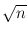Next: Bipartite Weighted Matchings and Up: Graph Algorithms Previous: Minimum Cut ( min_cut   Contents   Index

# Maximum Cardinality Matchings in Bipartite Graphs ( mcb_matching )

A matching in a graph G is a subset M of the edges of G such that no two share an endpoint. A node cover is a set of nodes NC such that every edge has at least one endpoint in NC. The maximum cardinality of a matching is at most the minimum cardinality of a node cover. In bipartite graph, the two quantities are equal.

 list MAX_CARD_BIPARTITE_MATCHING(graph& G) returns a maximum cardinality matching. Precondition G must be bipartite. list MAX_CARD_BIPARTITE_MATCHING(graph& G, node_array& NC) returns a maximum cardinality matching and a minimum cardinality node cover NC. The node cover has the same cardinality as the matching and hence proves the optimality of the matching. Precondition G must be bipartite. bool CHECK_MCB(const graph& G, const list& M, const node_array& NC) checks that M is a matching in G, i.e., that at most one edge in M is incident to any node of G, that NC is a node cover, i.e., for every edge of G at least one endpoint is in NC and that M and NC have the same cardinality. The function writes diagnostic output to cerr, if one of the conditions is violated. list MAX_CARD_BIPARTITE_MATCHING(graph& G, const list& A, const list& B) returns a maximum cardinality matching. Precondition G must be bipartite. The bipartition of G is given by A and B. All edges of G must be directed from A to B. list MAX_CARD_BIPARTITE_MATCHING(graph& G, const list& A, const list& B, node_array& NC) returns a maximum cardinality matching. A minimal node cover is returned in NC. The node cover has the same cardinality as the matching and hence proves the maximality of the matching. Precondition G must be bipartite. The bipartition of G is given by A and B. All edges of G must be directed from A to B.

We offer several implementations of bipartite matching algorithms. All of them require that the bipartition (A, B) is given and that all edges are directed from A to B; all of them return a maximum cardinality matching and a minimum cardinality node cover. The initial characters of the inventors are used to distinguish between the algorithms. The common interface is

   list<edge> MAX_CARD_BIPARTITE_MATCHING_XX(graph& G,
const list<node>& A,
const list<node>& B,
node_array<bool>& NC,
bool use_heuristic = true);

where XX is to be replaced by either HK, ABMP, FF, or FFB. All algorithms can be asked to use a heuristic to find an initial matching. This is the default.

HK stands for the algorithm due to Hopcroft and Karp . It has running time O(m).

ABMP stands for algorithm due to Alt, Blum, Mehlhorn, and Paul . The algorithm has running time O(m). The algorithm consists of two major phases. In the first phase all augmenting paths of length less than Lmax are found, and in the second phase the remaining augmenting paths are determined. The default value of Lmax is 0.1. Lmax is an additional optional parameter of the procedure.

FF stands for the algorithm due to Ford and Fulkerson . The algorithm has running time O(nm) and FFB stands for a simple and slow version of FF. The algorithm FF has an additional optional parameter use_bfs of type bool. If set to true, breadth-first-search is used in the search for augmenting paths, and if set to false, depth-first-search is used.

Be aware that the algorithms _XX change the graph G. They leave the graph structure unchanged but reorder adjacency lists (and hence change the embedding). If this is undesirable you must restore the original order of the adjacency lists as follows.

   edge_array<int> edge_number(G); int i = 0;
forall_nodes(v,G)